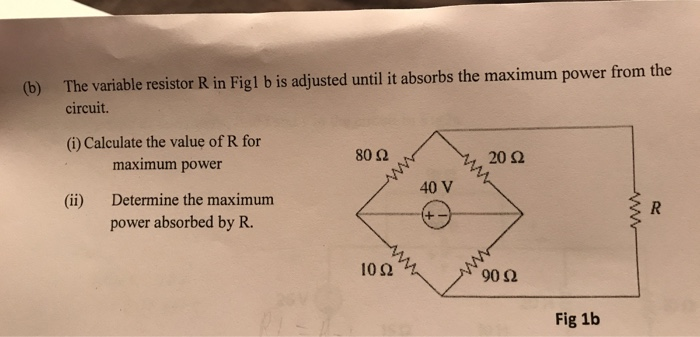### Create an Account

Already have account?

### Forgot Your Password ?

Home / Questions / The variable resistor R in Fig1 b is adjusted until it absorbs the maximum power from the ...

# The variable resistor R in Fig1 b is adjusted until it absorbs the maximum power from the b circuit i Calculate the valu The variable resistor R in Fig1 b is adjusted until it absorbs the maximumThe variable resistor R in Fig1 b is adjusted until it absorbs the maximum power from the (b) circuit. (i) Calculate the value of R for maximum power 20 Ω 80 Ω 40 V Determine the maximum (ii) power absorbed by R ww 10Ω 90 Ω Fig 1b ww

Jul 13 2020 View more View Less

#### Answer (Solved)Subscribe To Get Solution